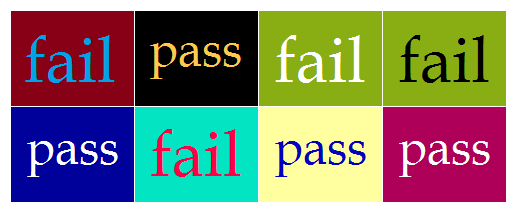# How to Calculate Color Contrast from RGB Values

## Principles of Good Web Design

 Enter a number from 0 to 255 for each color below. Background Color Text Color Red Red Green Green Blue Blue Can you read this easily?

When choosing the colors for text and background on your website, it is important to choose pairs of colors that have enough contrast between them. In web design, total color contrast has two components: hue difference (aka color difference), and light/dark difference (aka brightness difference). The higher the contrast between the text and background, the easier it is to read a webpage.

In web programming, a color is defined by the amount of red, green, and blue it contains. The RGB value of a color is a set of three numbers, each one ranging from 0 to 255 (or 00 to FF in hexadecimal). For example, the RGB value of a light yellow is (255, 248, 150).

Web designers use equations developed by the World Wide Web Consortium (W3) to calculate color contrast. To use these equations, you must know the RGB values of the text and background colors. These formulas and the acceptable contrast thresholds are explained below. You can also use the handy calculator on the left to compute color contrast. Just input the RGB values for the background and text colors.

## Calculating Brightness Difference

For ease of computation, let us call the red, green, and blue values of the text color R1, G1, and B1, respectively. And for the background color, let us call the RGB values R2, G2, and B2. The brightness index of the text color is determined by the equation

( 299R1 + 587G1 + 114B1 )/1000.

Likewise, the brightness index of background color is

( 299R2 + 587G2 + 114B2 )/1000.

The brightness difference is simply the difference between the two indices. So long as the brightness difference is greater than 125, the colors have sufficient light/dark contrast. For example, suppose the text color is dark brown with R1 = 60, G1 = 30, and B1 = 20. And suppose the background color is light blue with R2 = 180, G1 = 200, and B1 = 255. The brightness of the text color is

( 299*60 + 587*30 + 114*20 )/1000
= 38.

The brightness of the background color is

( 299*180 + 587*200 + 114*255 )/1000
= 200.

Since 200 - 38 = 162, these colors have sufficient light/dark contrast.

## Calculating Color Difference

The formula for the color difference between the text and background is

| R1 - R2 | + | G1 - G2 | + | B1 - B2 |

If the color difference is greater than 500, then the hue contrast is sufficient. If the color difference is 500 or less, it means the hues are too similar. Let us compute the hue difference for dark brown text over a light blue background:

| 60-180 | + | 30-200 | + | 20-255 |
= 120 + 170 + 235
= 525

Since this is larger than 500, we have sufficient hue contrast.

## Total Color Contrast

W3 deems that text and background colors have sufficient contrast when the brightness difference is over 125 AND the hue difference is over 500. If one of the two tests fails, the colors do not have sufficient contrast. The image below shows various combinations of text and background color. The most readable combinations pass both the brightness difference and color difference tests. The combos that are more difficult to read have failed one or both tests.